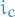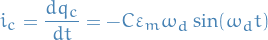# Fields

## Equations / Laws

### Coulomb's Law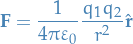1

where permittivity constant of free space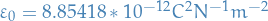### Electric Field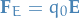where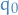is the test charge,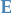is the electric field at that point.

### Magnetic Field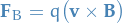### Lorentz Force

This is just writing the magnetic and electric in a single equation.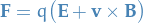### Electric Flux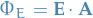### Gauss's Law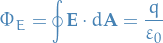for Gaussian surfaces, i.e. general closed surface with all surface elements pointing outwards.

### Potential Difference as work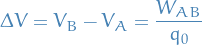I.e. potential-difference is simply work used on a test-charge.

### Potential Energy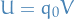### Potential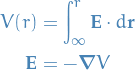### Ampere's Law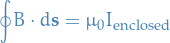### Biot-Savarts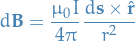where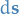is the vector whose magnitude is the length of the differential element of the wire in the direction of conventional current.

Thus,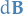is tangential to the surface of the wire, perpendicular to the current-flow (following the right-hand rule).

### Dipole moment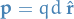2

where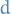is the distance between charges and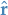is the dipole axis.

### Continuous Charge Distribution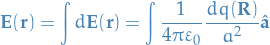where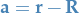### Torque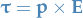Induced emf in a conducting loop is given by the rate of change of magnetic flux through the loop, i.e.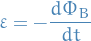### Lenz's Law

The induced current has a direction s.t. the magnetic field due to this current opposes the change in the magnetic field that caused it.

### Capacitor

#### Charge stored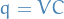## Definitions

### Electric dipole

Two charges of different charge separated by a fixed distance.

## Examples

### AC

Electromagnetic force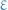is given by: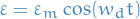The resulting current is: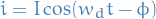where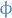is the phase-difference betweenand.

#### How-to

1. Setup the differential equation for charge wrt. time, using Kirchoff's Law and the fact that the potential difference across all components need to sum to the potential difference across the entire circuit.
2. Solve said differential equations.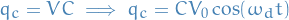Thus, "current" across the capacitor